# Multi-Objective Control Optimization for Congestion Avoidance in Computer Networks

Multi-Objective Control Optimization for Congestion Avoidance in Computer Networks
Faculty of Electrical Engineering, West Pomeranian University of Technology, Sikorskiego 37, Szczecin 70-313, Poland

Corresponding Author Email:
slawomir.grzyb@zut.edu.pl
Page:
39-44
|
DOI:
https://doi.org/10.18280/mmc_a.931-406
Received:
23 August 2020
|
Accepted:
6 December 2020
|
Published:
31 December 2020
| Citation

OPEN ACCESS

Abstract:

Effective congestion control is an issue strongly impacting basic features demanded from modern network environment as reliability, high and stable throughput, and low delays. These characteristics define the quality of communication channels. Optimizing network nodes configuration for only one of mentioned features, can exacerbate other parameters. This paper focuses on avoiding and alleviating network congestions using multi-objective optimization for gain setting of used controllers. Unlike in other presented approaches, in this case the non-stationary, discrete, dynamical model is discussed. The significant advantage of this approach is in the better reflection of the real environment conditions, where the transmission delay is floating. As the further development of the control strategy, the controller with the memory of previous steps have been deployed. Such control strategy mitigates the unfavorable impact of extended delays. Both proposed control strategies tune the presented model of communication channel to alleviate the results of sudden, unexpected network state changes. It is obtained by maximization of available bandwidth usage combined with minimization of buffer utilization. This supports avoiding undesirable congestion effects like packet dropping, retransmissions, high delay, and low network throughput.

Keywords:

channel model, discrete-time systems congestion avoidance, multi-objective optimization

1. Introduction

Congestion management in communication nodes plays the key role in maintaining efficient and reliable communication channels. Many approaches leading to alleviate network congestion effects has been presented in the literature [1-5]. This phenomenon strongly affects even different sorts of grids. The literature presents the progress in research in the field of power networks as in data exchange networks [1, 2]. Network environment can be finally decomposed into a system of many single bidirectional communication channels interfering with each other and competing for available resources [6, 7]. Such decompilation brings also the simplification into construction of different models of network segments. The occurrence of the bottlenecks in communication networks is connected both with limited interlinks bandwidth as with finite hardware resources of network nodes involved in the packets transmission . To mitigate the effects of blockage formation, variety methods and algorithms are developed and proposed, like sliding-mode algorithm [3, 8], fuzzy logic  and others [10-12]. Because of numerous constraints, like model simplification or computing consumption shrinking, these models have parameters constant in time. This paper presents the development of the non-stationary, discrete dynamical system model of data exchange communication channel. It was introduced in the paper  and further developed and tested. The discussed model relays on implementing time-varying delay, unlike in other approaches. It results in the change of system parameters, being the source of the model non-stationarity. The advantage of its implementation appears in the better reflection the real networking environments . In the previous research the usage of a piece-wise affine (PWA) algorithm have been proposed to this model [15, 16]. This method is widely due to the utilized in control algorithms [17, 18]. The application of this approach in nonlinear systems is discussed also by Orłowski . Stability of these systems is wider discussed in papers [17, 20-22].

The main gaol of the research presented in this paper is to control the length of outgoing packet queue to ensure both maximal available throughput utilization and minimal egress buffer utilization. For these colliding goals, two quality indexes have been introduced. The first one is monitoring the egress buffer occupancy of the congested node. The second one in focused on estimating the level of non-utilized available egress bandwidth. Optimizing these given functions, we find a set of optimal solutions. Multi-objective optimization using Non-dominated Sorting Genetic Algorithm (NSGA II) is used to find these solutions.

The same optimization approach has been applied for two control strategies and collated for the comparison purpose. Both these strategies are discussed in consequent chapters with the computation results.

In the first section of the paper presents non-stationary, discrete, dynamical model. It is followed by description of general issues of taken congestion modelling. Then two control strategies chosen for presented model are discussed. The summary of taken considerations and calculations is illustrated in numerical example.

2. Mathematical Model of the Network

The network communication channel model taken into consideration in this paper is like in [13, 15]. It’s a non-stationary, discrete dynamical model with the transmission delays varying in time. Considered fragment of network communication channel consists of a data source sending packets towards the destination. On their way, packets are passing through the certain number of active nodes, called intermediate nodes. These intermediate nodes introduce delays in packet transmission. There is a distinguished node among the others, called congested node (CN). This one acts in different way and can be considered as the weakest link in the nodes chain. Implementing congestion control into this node, leads to increase bandwidth utilization and efficient buffer occupancy. Available bandwidth is expressed as the number of packets that can potentially be sent from CN. Conditions meeting these requirements are following:

$0\le h\left( k \right)\le d\left( k \right)\le {{d}_{\max }}$         (1)

$0\le u\left( k \right)\le {{u}_{\max }}$       (2)

where:

h(k) - the number of packets successfully transmitted from the congested node toward the destination in time k.

d(k) - available bandwidth for CN in time k.

y(k) - queue length of CN in time k.

dmax, umax - maximum number of packets that can be sent towards destination, congested nodes respectively.

u(k) - number of packets requested by congested node from the source.

A full model of the communication channel in vector-matrix notation can be written in the following way:

\begin{align} & \mathbf{x}\left( k+1 \right)= \left[ \begin{array}{*{35}{l}} {{q}_{1}}\left( k \right) & 0 & 0 & \cdots & 0 \\ {{{\bar{q}}}_{1}}\left( k \right) & {{q}_{2}}\left( k \right) & 0 & \cdots & 0 \\ 0 & {{{\bar{q}}}_{2}}\left( k \right) & {{q}_{3}}\left( k \right) & 0 & \vdots \\ \vdots & \ddots & \ddots & \ddots & 0 \\ 0 & \cdots & 0 & {{q}_{n-1}}\left( k \right) & 1 \\\end{array} \right]\mathbf{x}\left( k \right)+\left[ \begin{matrix} 1 \\ 0 \\ \vdots \\ 0 \\ 0 \\\end{matrix} \right]u\left( k \right)+\left[ \begin{matrix} 0 \\ 0 \\ \vdots \\ 0 \\ -1 \\\end{matrix} \right]h\left( k \right) \\ \end{align}            (3)

$y\left( k \right)=\left[ \begin{matrix} 0 & 0 & \cdots & \begin{matrix} 0 & 1 \\\end{matrix} \\\end{matrix} \right]\mathbf{x}\left( k \right)$           (4)

where:

xj(k) - the number of packets accumulated in an intermediate node j at time-step k.

qj(k) - queuing factor.

{{q}_{j}}\left( k \right)=\left\{ \begin{align} & 0\text{ - transmission} \\ & 1\text{ - congestion} \\ \end{align} \right.

${{\bar{q}}_{j}}\left( k \right)=1-{{q}_{j}}\left( k \right)$.

y(k) - congested node’s queue length at time-step k.

3. Congestion Control Strategy

Network environmental resistance on the formation of the flow blockages strongly depends on buffers utilization level of network nodes participating in data exchange process. When a buffer is filled up, the node is not able to accept any incoming datagrams. In this state incoming packets must be dropped. That leads to retransmission attempts by the upper network layers of terminals involved in data exchange process. It finally results in increasing network occupancy and decreasing effective network throughput. On the other hand, in case of sudden and unpredicted throughput rise, a buffer should store enough data, not to be completely emptied before new data portion would feed it. That means, that a safe margin of utilization must be maintained in order not to completely empty or not to overflow buffers during unexpected sudden changes in the network.

To fulfill these requirements of the effective bandwidth utilization and buffer occupancy, two two-dimensional controllers have been proposed. Both use a momentary value of available bandwidth d(k) for determining reference buffer occupancy level, but the process of control signal calculation differs significantly.

The first control strategy is shown in Figure 1.

## 1.pngFigure 1. Block diagram of the first control strategy

In this case, a linear relationship between u(k) and queue length error:

$u\left( k \right)={{z}_{1}}\left( {{y}_{r}}-y(k) \right)$          (5)

where:

${{y}_{r}}$ - reference queue length of congested node.

z1 - linear gain of the first controller.

Another linear relation between reference value yr and available bandwidth d(k) is presented below:

${{y}_{r}}(k)={{z}_{2}}d(k-1)$          (6)

where:

z2 - linear gain of the second controller.

Substituting Eq. (6) in (5), we can finally formulate following equation:

$u\left( k \right)={{z}_{1}}\left( {{z}_{2}}d(k-1)-y(k) \right)$             (7)

The advantage of the utilization of available bandwidth as a component of error signal is deeper discussed by Grzyb and Orłowski . Since the proposed model has variable delay, we can expect that the minimal time-steps difference between the control signal adjustment and the observed result of this change is equal to the system order. Such delay combined with the dynamics of available bandwidth level is highly demanding from the perspective of the optimal control.

As the response to this fact, the second control strategy has been proposed. Respecting (6), the second approach delivers different method of an error signal computing. The concept of this strategy is presented in Figure 2.

## 2.pngFigure 2. Block diagram of the second control strategy

In this approach, there is an additional block called Work In Progress (WIP). This block impacts directly the value of an error signal. WIP block accumulates the knowledge about ordered packets, which due to the delay didn't reach CN yet and the delivery phase is in progress. This information is used for the better adjustment of the control signal, because these packets are added to the value of y(k). Now the algorithm knows how many packets are in delivery and accommodates the control signal, which now is represented by the following equation:

$u\left( k \right)={{z}_{1}}\left( {{z}_{2}}d(k-1)-\left( y(k)+\sum\limits_{i=k-1-\tau }^{k-1}{u(i)} \right) \right)$            (8)

where:

τ - minimal latency following from the assumed system order.

Since the model in non-stationary, and the delay is varying in time, it can never be lower than the system order.

For both control strategies, wanted z1 and z2 we can define as vector coordinates:

$\mathbf{z}=\left[ \begin{matrix} {{z}_{1}} & {{z}_{2}} \\\end{matrix} \right]$             (9)

To adjust the control to the selected design requirements, two cost functions need to be defined. It can be described as follows:

${{J}_{1}}(\mathbf{z})=\sum\limits_{k=1}^{N}{\left( d(k)-h(k,\mathbf{z}) \right)}$            (10)

${{J}_{2}}(\mathbf{z})=\sum\limits_{k=1}^{N}{y(k,\mathbf{z})}$                 (11)

Function (10) defines the penalty for not utilizing available bandwidth in time horizon. It is calculated as the difference between the number of packets that could be potentially sent due to available bandwidth and the number of packets that have been successfully sent. The sum (11) is representing cumulated buffer occupancy. Lowering this value enables to use the free buffer space by other data streams between the same or different participants.

Given that the system is described with model (3)-(4), relation (7) and defined cost functions (10), (11), the optimal vector (9) coordinates can be determined solving the following multi-objective optimization problem:

$\underset{\mathbf{z}}{\mathop{\min }}\,{{J}_{1}},{{J}_{2}}$         (12)

4. Simulation Results

In order to illustrate proposed control systems, the following discrete non-stationary linear model is assumed. In this example, the numerical simulation of the communication channel with time-varying delay is made. The analysis subject is the length of the outgoing packet queue in the congested node. To fit model delays to those existing in real computer networks an order of system is assumed to be 30. For the purposes of simulation, it is assumed that the system can be described by the following discrete non-stationary linear model:

\begin{align} & \mathbf{x}\left( k+1 \right)=\mathbf{A}\left( k \right)\mathbf{x}\left( k \right)+\mathbf{B}\left( k \right)u\left( k \right)+\mathbf{F}\left( k \right)h\left( k \right) \\ & y\left( k \right)=\mathbf{C}\left( k \right)\mathbf{x}\left( k \right) \end{align}       (13)

where:

\begin{align} & \mathbf{A}\left( k \right)={{\mathbf{A}}_{\kappa }}, \\ & \mathbf{B}\left( k \right)=\mathbf{B}={{[1,0,0,...,0]}^{T}}, \\ & \mathbf{C}\left( k \right)=\mathbf{C}=[0,0,...,0,1], \\ & \mathbf{F}\left( k \right)=\mathbf{F}={{[0,0,...,0,1]}^{T}} \\ & \kappa =floor\left( rem\left( \frac{k}{\varepsilon },0,04 \right) \right) \\ \end{align}             (14)

${{\mathbf{A}}_{0}}=\left[ \begin{matrix} 0 & 0 & 0 & 0 & 0 & 0 \\ 1 & 0 & 0 & 0 & 0 & 0 \\ 0 & 1 & 0 & 0 & 0 & 0 \\ \vdots & \ddots & \ddots & \ddots & \ddots & \vdots \\ 0 & 0 & 0 & 1 & 0 & 0 \\ 0 & 0 & 0 & 0 & 1 & 1 \\\end{matrix} \right]$           (15)

Matrix A0 is like that in the paper  and represents a model of the communication channel without congestion in the intermediate nodes. Matrix A1 mirrors the state when blockage occurs in the 7th intermediate node. It is based on matrix A0 with following differences: element A1(7,7)=1 and element A1(8,7)=0. The system state, in which node 21st can’t forward packets is mapped by a matrix A2. It’s built on A0 pattern, taking into consideration that A2(21,21)=1 and A2(22,21)=0. Matrix A3 represents a model of congestion in the 14th node. Like previous values it’s based on A0 with two different elements: A3(14,14)=1 and A3(15,14)=0. Vectors B(k), C(k), and F(k) are constant in time.

For purposes of numerical simulation of model (13) - (15) and both control strategies, it has been assumed that:

dmax=umax=100 packets per sample, the maximum buffer capacity of the congested node ymax=5000 packets and WIP delay τ=30. Sampling period is 10[ms] and all buffers in the communication channels are empty. Assumed bandwidth d(k) currently available for the congested node towards destination is shown in Figure 3.

## 3.pngFigure 3. Available outgoing bandwidth for the CN

For the computation purpose it is assumed: population size and number of generations both equals 1000.

On the basis of given model (3)-(4), defined cost functions (10), (11) and relations (7) and (8), two following sets of optimal solutions have been obtained using multicriteria optimization with NSGA II algorithm. The results are shown in Figure 4.

## 4.pngFigure 4. Pareto front obtained by multi-objective optimization

We can observe the definite improvement of the results for the control strategy with WIP in almost all observed scope. For each Pareto’s front, there are three example samples distinguished in Figure 4. These examples are presented in Table 1, collated with J1 and J2 values and corresponding to coordinates of the vector z:

Table 1. Chosen sets of calculated results

 No. J1 J2 z1 z2 11 6.2000e+02 1.4515e+06 8.4378e+02 4.7195e+01 12 3.4024e+03 9.1741e+05 3.8634e-01 2.2550e+01 13 4.9738e+03 5.0434e+05 3.3246e-02 3.3981e+01 21 6.2000e+02 1.5706e+06 2.1207e+03 1.3140e+02 22 3.4124e+03 5.0515e+05 9.4955e+00 3.4339e+01 23 4.9652e+03 4.3526e+05 6.5137e+00 3.0838e+01

Appling controllers parameters from Table 1, the queue length of congested node for both control strategies is illustrated in Figure 5.

## 5.png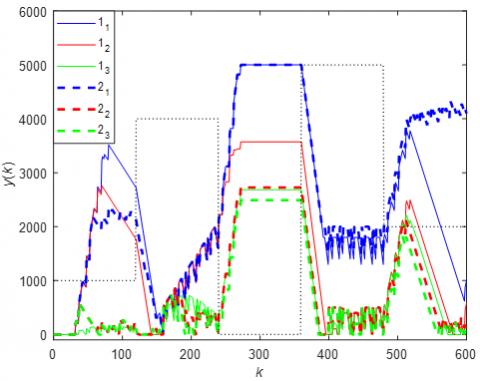Figure 5. Congested node’s queue length for different controller parameters z for both control strategies

There is also an auxiliary black dotted line in the above Figure 5. It represents calibrated d(k) as a reference. In Table 1 we can see that for the same value of J1 for point 11 and 12, the optimal value is of J2 is higher for the strategy with memory.

Figure 5 delivers the information that for the control strategy with WIP, controller tries to avoid an excessive buffer draining after the sudden bandwidth decrease in time-step 480.

In case of obtained z vectors, it can be seen that coordinates set, which ensures lower buffer utilization, can’t supply sufficient packet number to satisfy available bandwidth, and vice versa. In order to present the full relation spectrum, in the proposed range, between vector (9) and functions (10) and (11) for both control strategies, four 3D surfaces are presented.

Figures 6 and 7 present the cost functions for the control strategy without WIP. Figures 8 and 9 illustrate results for the second control strategy with WIP.

The relation between vector z coordinates and function J1 for (7) is presented in Figure 6, whereas the relation between vector z coordinates and function J2 for (7) is presented in Figure 7. The relation between vector z coordinates and function J1 for (8) is presented in Figure 8.

Finally. the relation between vector z coordinates and function J2 for (8) is presented in Figure 9.

## 6.png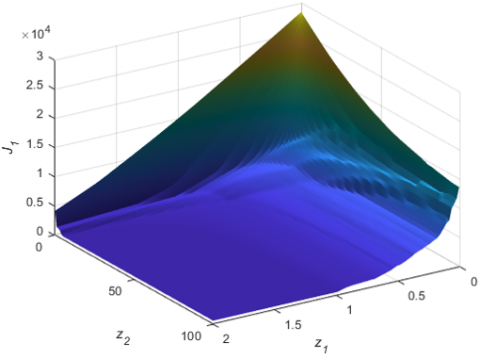Figure 6. Cost function J1 vs. controller gains for the control strategy without WIP

## 7.png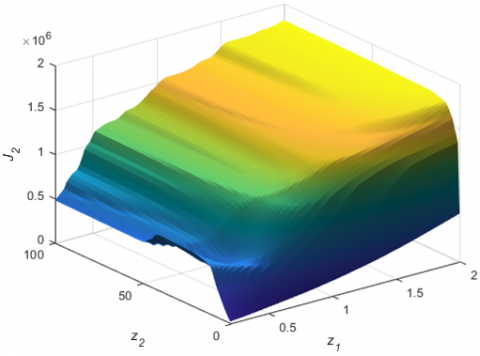Figure 7. Cost function J2 vs. controller gains for the control strategy without WIP

In Figures 6 and 7, we observe an approximate peak increase of unused available bandwidth if z1 and z2 values tend to 0. These coordinates reflect the gain of the controller and the level of the reference signal, what makes the explanation of this phenomenon. If we don't deliver any packets to the CN, we can expect the high level of underutilization of the available bandwidth. For the strategy with WIP we observe the better response to the d(k) variation, what appears as the lower level of surface deflections.

Figures 8 and 9 present the level of the CN’s egress buffer utilization. In this case the second control strategy with WIP delivers smother surface together with the lower maximal values and faster descent of J1 along with z1 and z2 decrease.

## 8.png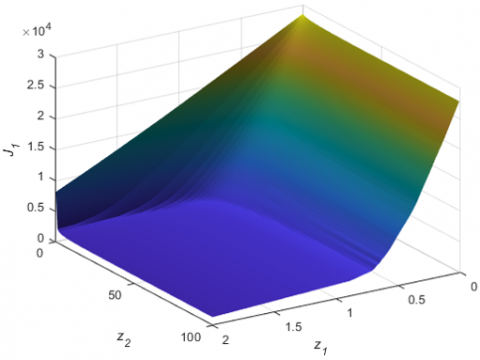Figure 8. Cost function J1 vs. controller gains for the control strategy with WIP

## 9.png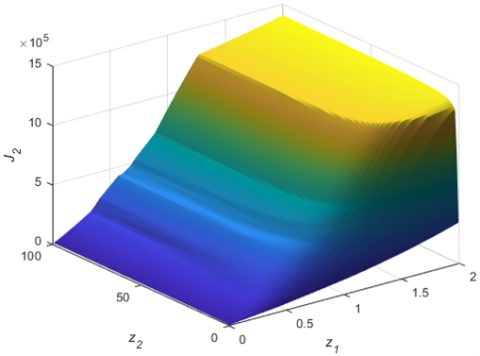Figure 9. Cost function J2 vs. controller gains for the control strategy with WIP

5. Conclusions

The paper covers the use of multi-objective optimization in purpose of congestion avoidance in data exchange networks. This goal is achieved by controlling egress buffer utilization in active network nodes. In order to ensure both effective bandwidth utilization and minimization of buffer occupancy, double controller have been proposed. Cost functions have been defined with the respect the design objectives. Two control strategies have been introduced and subjected to optimization process. Pareto fronts are calculated. Presented numerical examples confirm that given cost functions allow the model functioning in expected manner. Control strategies comparison prioritize the approach of the deployment the strategy with the memory of previously ordered packets. The concept of the usage WIP module for the non-stationary system with high and time-varying delay brings the control quality improvement.

This research can be extended further by taking account and investigate the impact of uncertainty, disturbances and possibly include adaptation of the model to the real network environment.

References

 Hazara, J., Sinha, A.K. (2007). Congestion management using multiobjective particle swarm optimization. IEEE Trans. Power Syst., 22(4): 1726-1734. http://dx.doi.org/10.1109/TPWRS.2007.907532

 Panigrahi, B.K., Pandi, V.R. (2009). Congestion management using adaptive bacterial foraging algorithm. Energy Conversion and Management, 50(5): 1202-1209. http://dx.doi.org/10.1016/j.enconman.2009.01.029

 Ignaciuk, P., Bartoszewicz, A. (2011). Discrete-time sliding-mode congestion control in multisource communication networks with time-varying delay. IEEE Trans. on Control Systems Technology, 19(4): 852-867. http://dx.doi.org/10.1109/TCST.2010.2056690

 Danielson, C., Borrelli, F., Oliver, D., Anderson, D., Phillips, T. (2013). Constrained flow control in storage networks: Capacity maximization and balancing. Automatica, 49(9): 2612-2621. http://dx.doi.org/10.1016/j.automatica.2013.05.014

 Grzyb, S., Orłowski, P. (2013). Mechanizmy powstawania zatorów i blokad komunikacyjnych w sieciach o zmiennych w czasie parametrach. Pomiary Automatyka Kontrola, 59(7): 704-707.

 Sun, H.J., Wu, J.J., Ma, D., Long, J.C. (2014). Spatial distribution complexities of traffic congestion and bottlenecks in different network topologies. Applied Mathematical Modelling, 38(2): 496-505. http://dx.doi.org/10.1016/j.apm.2013.06.027

 Jiang, N., Becker, D.U., Michelogiannakis, G., Dally, W.J. (2012). Network congestion avoidance through speculative reservation. IEEE International Symposium on High-Performance Comp Architecture, New Orleans, LA, pp. 1-12. http://dx.doi.org/10.1109/HPCA.2012.6169047

 Ignaciuk, P., Bartoszewicz, A. (2007). Linear quadratic optimal sliding mode controllers for a single virtual circuit in a connection-oriented communication network. Proceedings of the 13th IEEE/IFAC International Conference on Methods and Models in Automation and Robotics, Szczecin, Poland, pp. 121-128.

 Nyirenda, C.N., Dawou, D.S. (2007). Fuzzy logic congestion control in IEEE 802.11 wireless local area networks: A performance evaluation. AFRICON 2007, Windhoek, pp. 1-7. https://doi.org/10.1109/AFRCON.2007.4401645

 Guan, X.P., Yang, B., Zhao, B., Feng, G., Chen, C.L. (2007). Adaptive fuzzy sliding mode active queue management algorithms. Telecommunication Systems, 35: 21-42. http://dx.doi.org/10.1007/s11235-007-9040-6

 Masoumzadeh, S.S., Meshgi, K., Ghidari, S.S., Taghizadeh, G. (2011). FQL-RED: An adaptive scalable schema for active queue management. International Journal of Network Management, 21(2): 147-167. https://doi.org/10.1002/nem.755

 Nyirenda, C.N., Dawoud, D.S. (2006). Multi-objective particle swarm optimization for fuzzy logic based active queue management. IEEE International Conference on Fuzzy Systems, pp. 2231-2238. http://dx.doi.org/10.1109/FUZZY.2006.1682010

 Grzyb, S., Orłowski, P. (2013). Model matematyczny kanału komunikacyjnego z zatorem w sieciach o zmiennych w czasie parametrach. Pomiary Automatyka Kontrola, 59(11): 1151-1154.

 Grzyb, S., Orlowski, P. (2015). Congestion feedback control for computer networks with bandwidth estimation. 20th International Conference on Methods and Models in Automation and Robotics (MMAR), Miedzyzdroje, Poland, pp. 1151-1156. http://dx.doi.org/10.1109/MMAR.2015.7284041

 Grzyb, S., Orłowski, P. (2016). Feedback control system with PWA load dependent reference buffer occupancy for congestion control in computer networks. Przegląd Elektotechniczny, 92(4): 42-45. http://dx.doi.org/10.15199/48.2016.04.11

 Grzyb, S., Orlowski, P. (2014). Congestion control in computer networks: Application of piece-wise affine controller and particle swarm optimization. 19th International Conference on Methods and Models in Automation and Robotics (MMAR), Miedzyzdroje, Poland, pp. 834-838. http://dx.doi.org/10.1109/MMAR.2014.6957465

 Grieder, P., Kvasnica, M., Baotic, M., Morari, M. (2004). Low complexity control of piecewise affine systems with stability guarantee. Proceedings of the 2004 American Control Conference, Boston, MA, USA, 2: 1196-1201. http://dx.doi.org/10.23919/ACC.2004.1386735

 Orlowski, P. (2012). Effect of the partitions of approximate secant piece-wise affine model on control quality for non-linear, state-dependent system. Przegląd Elektrotechniczny, 88(10A): 69-73.

 Orłowski, P. (2011). Convergence of the discrete-time nonlinear model predictive control with successive time-varying linearization along predicted trajectories. Electronika ir Elektrotechnika, 113(7): 27-31. http://dx.doi.org/10.5755/j01.eee.113.7.607

 Besselmann, T., Lofberg, J., Morari, M. (2012). Explicit MPC for LPV systems: Stability and optimality. IEEE Transactions on Automatic Control, 57(9): 2322-2332. http://dx.doi.org/10.1109/TAC.2012.2187400

 Orłowski, P. (2012). Generalized feedback stability for periodic linear time–varying, discrete–time systems. Bulletin of the Polish Academy of Sciences: Technical Sciences - Polish Academy of Sciences, 60(1): 171-178. http://dx.doi.org/10.2478/v10175-012-0024-7

 Bemporad, A., Oliveri, A., Poggi, T., Storace, M. (2011). Ultra-fast stabilizing model predictive control via canonical piecewise afﬁne approximations. IEEE Trans. Automatic Control, 56(12): 2883-2897. http://dx.doi.org/10.1109/TAC.2011.2141410